Get instant live expert help with Excel or Google Sheets“My Excelchat expert helped me in less than 20 minutes, saving me what would have been 5 hours of work!”

#### Post your problem and you’ll get expert help in seconds.

Your message must be at least 40 characters
Our professional experts are available now. Your privacy is guaranteed.

# Bond price formula – Excelchat

Bonds essentially refer to a contract between an investor and a borrower.  It is a type of loan to be repaid at the end of the maturity period. Some bonds have annual or semi-annual coupons which are basically predetermined interest payments.  These coupons are incurred as additional cost of borrowing, and are paid to investors on a regular basis until the bond’s maturity.

This article will show us how to calculate the bond value or bond price for a zero coupon bond, an annual coupon bond and a semi-annual coupon bond.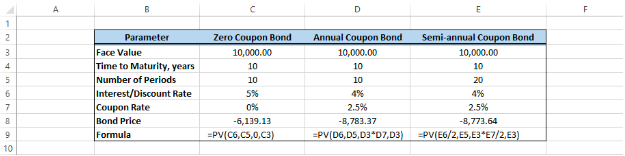Figure 1.  Final result:  Bond price formula

## Calculate bond price

Bond price is the current discounted value of a future cash flow.  In simple terms, a bond price is the sum of the present value of the principal payment and the interest payments.  In order to calculate the bond price, we can use the PV function.

## Syntax of PV function

`=PV(rate, nper, pmt, [fv], [type])`

• Rate  is the interest rate per period
• Nper is the total number of payment periods
• Pmt is the payment made each period and is fixed until bond maturity
• Fv is the future value at bond maturity
• Type is either 0 ((if payment is at the end of the period) or 1 (payment at the beginning of the period)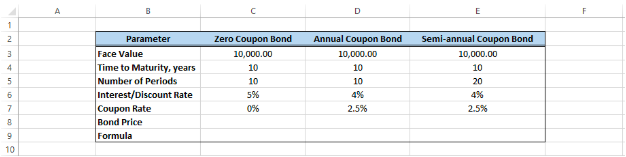Figure 2.  Sample data to calculate bond price

## Price of zero coupon bond

Our example is a ten-year bond with a face value of 10,000 and an interest rate of 5%.  In order to calculate the bond price with no coupons, we enter this formula in C8:

`=PV(C6,C5,0,C3)`

• Interest rate = 5%
• Number of periods = 10
• Payment made each period = 0 (zero coupons)
• Face value = 10,000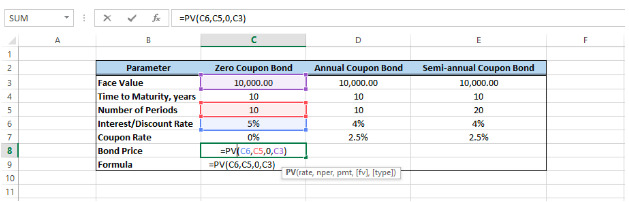Figure 3.  Bond price formula for zero coupons

The resulting bond price is -6,139.13.  In accounting, the negative sign means it is an outgoing cash flow.

## Price of annual coupon bond

There are bonds wherein the investors get a coupon each year.  In order to calculate the price of an annual coupon bond, we enter this formula in D8:

`=PV(D6,D5,D3*D7,D3)`

• Discount rate = 4%
• Number of periods = 10
• Payment made each period = 10,000 x 2.5%
• Face value = 10,000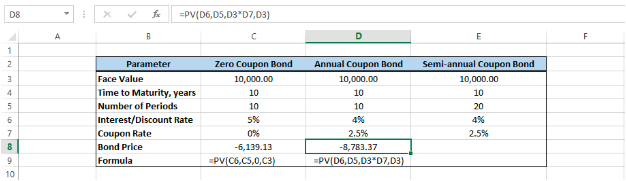Figure 4.  Bond price formula for annual coupon bond

As a result, the bond price is 8783.37.

Price of semi-annual coupon bond

There are also bonds that give out coupons twice in a year.  The formula for bond price is:

`=PV(E6/2,E5,E3*E7/2,E3)`

• Discount rate = 4%/2 = 2%
• Number of periods = 10×2 = 20
• Payment made each period = 10,000 x 2.5%/2
• Face value = 10,000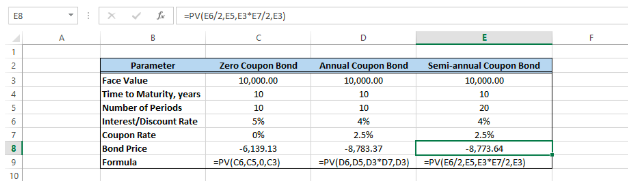Figure 5.  Bond price formula for semi-annual coupon bond

Note that for semi-annual coupons, we divide the discount rate and coupon rate by 2, and the number of periods is twice the number of years.  The resulting bond price is 8773.64.

## Instant Connection to an Excel Expert

Most of the time, the problem you will need to solve will be more complex than a simple application of a formula or function. If you want to save hours of research and frustration, try our live Excelchat service! Our Excel Experts are available 24/7 to answer any Excel question you may have. We guarantee a connection within 30 seconds and a customized solution within 20 minutes.